# Motion2

Cyclist started out of town at 19 km/h. After 0.7 hours car started behind him in the same direction and caught up with him for 23 minutes. How fast and how long went car from the city to caught cyclist?

Result

v2 =  53.7 km/h
s =  20.6 km

#### Solution:s = 19 t
s = v * 23/60
t = 0.7+23/60

s-19t = 0
60s-23v = 0
60t = 65

s = 24712 ≈ 20.583333
t = 1312 ≈ 1.083333
v = 123523 ≈ 53.695652

Calculated by our linear equations calculator.

Leave us a comment of example and its solution (i.e. if it is still somewhat unclear...):

Showing 0 comments:Be the first to comment!#### To solve this example are needed these knowledge from mathematics:

Need help calculate sum, simplify or multiply fractions? Try our fraction calculator. Do you have a linear equation or system of equations and looking for its solution? Or do you have quadratic equation? Do you want to convert length units?

## Next similar examples:

1. The cyclist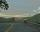The cyclist went from village to town. First half of journey went at 20 km/h. The second half of the journey, which mostly fell, went at 39 km/h. All journey took 88 minutes. Calculate the distance from the village to the town.
2. Cyclists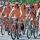Cyclist who rides at an average speed 16 km/h travels trip distance 10 min before the cyclist who rides at an average speed 11 km/h. What is the length of this cyclist trip(distance in km)?
3. TruckIn 7 hours started from town Krnov truck at speed 40 km/h. Passenger car started against it in 8 hours 30 minutes from the city of Jihlava at speed 70 km/h. Distance between this two cities is 225 km. At what time and at what distance from Krnov this two.
4. Two cars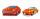From the town A to town B started two cars. The first at 7:00 at average speed 60 km per hour, the second at 10:00 at average speed 100 km per hour. The first car will not stay in B, and on the way back meet the second car at half way from A to B. At what.
5. TrainsFrom station 130 km away started passenger train and after 2.2 hours after the express train, which travels 37 km an hour more. Express train finish journey 7 minutes early. Calculate the average speed of this two trains.
6. Train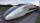A passenger train traveled for 2 hours 74 km. 3.1 hours after its departure started fast train and caught it on 186 km. How many km/h is different its average speeds?
7. Tourists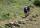From the cottage started first group of tourists at 10:00 AM at speed 4 km/h. The second started after them 47 minutes later at speed 6 km/h. How long and how many kilometers from the cottage will catch the first group?
8. Train from PragueFirst train from Prague started at 8:00 hour at 40 kilometers per hour. Train from Ostrava started at 9:20 at 80 km per hour. In how many hours and how far from cities with trains meet if the distance of cities is 400 km.
9. Train from BrnoFirst train started from Brno at 8 am at 60 km per hour. Second train started from Opava at 7 o'clock at the speed of 40 kilometers per hour. In how many hours and how far from cities trains meet when the distance of cities is 200 kilometers.
10. MotionFrom two different locations distant 232 km started against car and bus. The car started at 5:20 with average speed 64 km/h. Bus started at 7:30 with average speed 80 km/h. When they meet? How many kilometers went the bus?
11. Vacation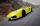Parents piggybacking children on vacation to its grandmother and grandfather at a city distant 150 kilometers. They agreed to meet halfway. Parents will be travel at 90 km/h, grandmother and grandfather at 60 km/h. Parents depart at 12:00 hours. When grand
12. Paul and Honza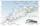Honza went to chat on a bike without a break 4.0 hours. Paul the same way without breaks 50 minutes longer. The difference between the speeds of the two boys was the one kilometer per hour. How fast was Paul, if you know that the speed of the boys did not.
13. Pedestrian up-down hill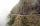Pedestrian goes for a walk first at plane at 4 km/h, then uphill 3 km/h. Then it is in the middle of the route, turns back and goes downhill at speed 6 km/h. Total walk was 6 hours. How many kilometers went pedestrian?
14. Scouts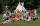The boys from scout group traveled 5 days distance 115 km. Every day walked 1.5 km less than the previous day. How many kilometers scouts walked in the first day?
15. The carThe car has traveled the distance between A and B for four hour. If we increased the average by 17 km/h the car travel this distance an hour earlier. Determine the initial speed of the car and the distance between A and B.
16. Forth and back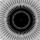Car goes from point A to point B at speed 78 km/h and back at 82 km/h. If went there and back at speed 81 km/h journey would take five minutes less. What is the distance between points A and B?
17. Journey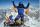The road from A to B measures 11.5 km. Firstly up the hill, then by level plane and then downhill. Tourist goes uphill at 3 km/h, on the plane 4 km/h and downhill 5 km/h. From point A to B went 2h 54 min back 3h 6 min. How long is the segment of level pla